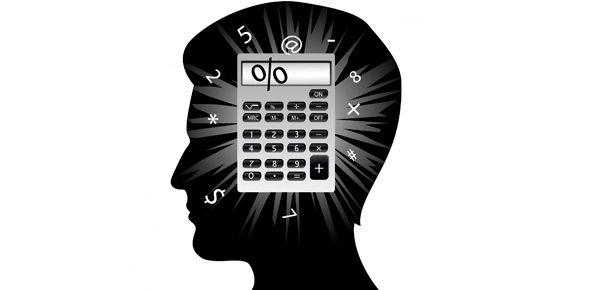# Timed Mental Mathematics(Fixed)

4 Questions | Total Attempts: 1575SettingsDo you think you can do mental math quickly? Are you smart enough to complete questions within 7 minutes? You can find out now.

Related Topics
• 1.
Solve for x.    (3+4)-2+x=10
• A.

X=5

• B.

X=4

• C.

X=7

• D.

X=3

• E.

X=6

• 2.
Solve for z.56=(17-9)+69-z
• A.

Z=19

• B.

Z=18

• C.

Z=21

• D.

Z=20

• E.

Z=16

• 3.
Combine Like terms.2a+3b-a-2b
• A.

4a+3b-a-2b

• B.

4a+1b-a

• C.

5a-a

• D.

1a+1b

• E.

6a+3b

• 4.
If p= 14, if which of the following statements are true?
• A.

30-p=15

• B.

4p=54

• C.

P+p+p+18=70

• D.

P-7+1467=1477

• E.

P= 35-(8+13)